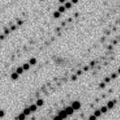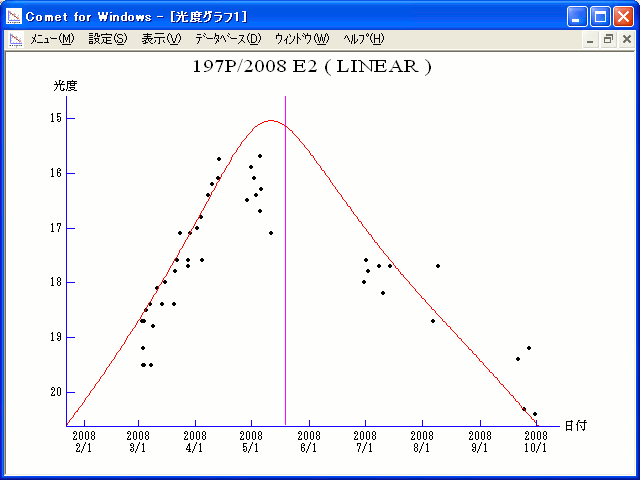# \$B%j%K%"WB@1(B

197P/LINEAR (2008)###\$B%W%m%U%#!<%k(B

 \$BId9f(B 197P/2008 E2 \$B8!=PF|(B 2008\$BG/(B3\$B7n(B3\$BF|(B \$B8!=P8wEY(B 18.7\$BEy(B \$B8!=P R. E. Hill (Catalina)

###\$B###\$B50F;MWAG(B

```Epoch 2008 May 14.0 TT = JDT 2454600.5
T 2008 May 19.01808 TT                                  Kobayashi
q   1.0604282            (2000.0)            P               Q
n   0.20311846     Peri.  188.79441     -0.26942972     +0.87816552
a   2.8661777      Node    66.38904     -0.85524467     -0.02952289
e   0.6300201      Incl.   25.55487     -0.44267841     -0.47744499
P   4.85
From 304 observations 2003 May 23-2013 Sept. 25, mean residual 0".7.
Nongravitational parameters A1 = +0.00, A2 = +0.0018.
```

###\$B@1?^(B###\$B8wEYJQ2=(B

```        m1 = 17.5 + 5 log\$B&\$(B + 10.0 log r
```##### \$B50F;MWAG\$O(BM.P.E.C. 2015-M77\$B\$K7G:\\$5\$l\$?\$b\$N\$G\$9!#(B \$B@1?^\$O%9%F%i%J%S%2!<%?(B Ver.8 (\$B%"%9%H%m%"!<%D(B) \$B\$G:n@.\$7\$?\$b\$N\$G\$9!#(B \$B8wEY%0%i%U\$O(BComet for Windows\$B\$G:n@.\$7\$?\$b\$N\$G\$9!#(B